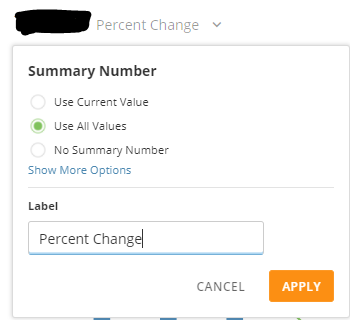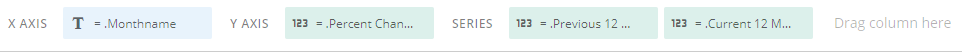# PoP Cards with Summary Number Showing the Total PoP Variance

I'm using the PoP cards to show monthly variances versus the same month in the prior year.  I'd like to have the summary number show the total variance as a percent increase/decrease across the full time period selected in the card.  For example, the default time period is YTD and is displayed monthly.  I'd like the summary number to show the total YTD change versus the same period in the prior year. Basically, the summary number should show the variance percentage, if you graphed by year instead of month.

What would be ideal is if the summary number were dynamic so it always shows the total for whatever time period the user selects, even if it's across years.

Is that possible?

Thanks,

Nathan

•CoachI have actually created this card in our environment. It's showing this year by month over last year (same period) I have the variance % trending over each month and the total variance for the year is achieved by having the BeastMode for the variance % set to "Use All Values".  As shown belowHere's a generalized version of my BeastMode formula. You should be able to tailor this to your needs:

CASE WHEN
(COUNT(CASE WHEN
`Date Field` IS NOT NULL and
`Date Field` < DATE_SUB(DATE_FORMAT(CURDATE(), '%Y-%m-01'),interval 11 month) AND
`Date Field` >= DATE_SUB(DATE_FORMAT(CURDATE(), '%Y-%m-01'),interval 23 month) AND
`Date Field` < DATE_SUB(CURDATE(), interval 1 year)
THEN `Field to Count`
END)) = 0
THEN 0
ELSE

(COUNT(CASE WHEN
`Date Field` IS NOT NULL and
`Date Field` >= DATE_SUB(DATE_FORMAT(CURDATE(), '%Y-%m-01'),interval 11 month) AND
`Date Field` <= CURDATE()
THEN `Field to Count`

END)
/
COUNT(CASE WHEN
`Date Field` IS NOT NULL and
`Date Field` < DATE_SUB(DATE_FORMAT(CURDATE(), '%Y-%m-01'),interval 11 month) AND
`Date Field` >= DATE_SUB(DATE_FORMAT(CURDATE(), '%Y-%m-01'),interval 23 month) AND
`Date Field` <= DATE_SUB(CURDATE(), interval 1 year)
THEN `Field to Count`
END))
- 1

END

The purpose of the first case is to eliminate any errors from a divide by 0 for previous year count.

This is basically doing the (Current Year Count) / (Previous Year Count) - 1 which gives us variance or % change.

Let me know if you have any questions.

**Say "Thanks" by clicking the thumbs up if this post helped you.

• Thanks for the quick reply.  Your suggestion conceptually works, but it doesn't seem to work for my use case.  I'm using one of the new Period Over Period chart types and have the date set to Year to Date, by Month.  I can see the variance for each month in the chart and could see the YTD YoY variance if I graph it by year.  What I'm hoping to do is show it by month in the chart and then have the summary number show it at the aggregate level.  I'm thinking it doesn't work since the card is set to Year to Date, so Domo doesn't view the prior year data as being part of the date range from the summary number perspective.

•CoachAhh, I see. I'm actually doing PoP using a Line + Grouped Bar.My X-Axis is month names using:

MONTHNAME(`Date Field`)

Y-Axis is the Variance BeastMode from earlier.

And 2 Series fields

Previous 12 Months:

COUNT(CASE WHEN `Date Field` IS NOT NULL and `Date Field` < DATE_SUB(CURDATE(), interval 12 month) AND `Date Field` >= DATE_SUB(DATE_FORMAT(CURDATE(), '%Y-%m-01'),interval 23 month)  THEN `Field to Count` END)

Current 12 Months:

COUNT(CASE WHEN `Date Field` IS NOT NULL and `Date Field` >= DATE_SUB(DATE_FORMAT(CURDATE(), '%Y-%m-01'),interval 11 month) THEN `Field to Count`   END)

This way it breaks it across months, you have two columns, one for this year and previous year and your variance line across the graph.

Maybe this will work for you as well?

• I'm having the same issue - hoping there's a solution to this!

This discussion has been closed.